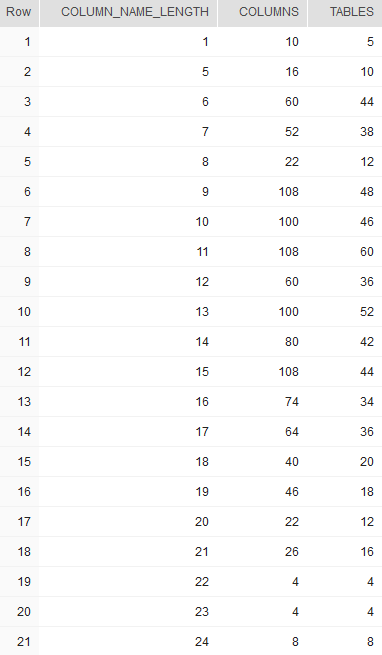## Get column name length distribution in Snowflake

Article for:

Query below returns distribution of column name lengths (number of characters).

## Query

``````select length(c.column_name) as column_name_length,
count(*) as columns,
count(distinct t.table_schema || '.' ||  t.table_name) as tables
from information_schema.tables t
inner join information_schema.columns c on
c.table_schema = t.table_schema and c.table_name = t.table_name
where table_type = 'BASE TABLE'
group by length(c.column_name)
order by length(c.column_name);
``````

## Columns

• column_name_length - lenght in characters of column name
• columns - number of columns with this length
• tables - number of tables that have columns with this name length

## Rows

• One row represents one name lenght (number of characters)
• Scope of rows: each column length that exists in a database
• Ordered by length ascending (from 1 to max)

## Sample results